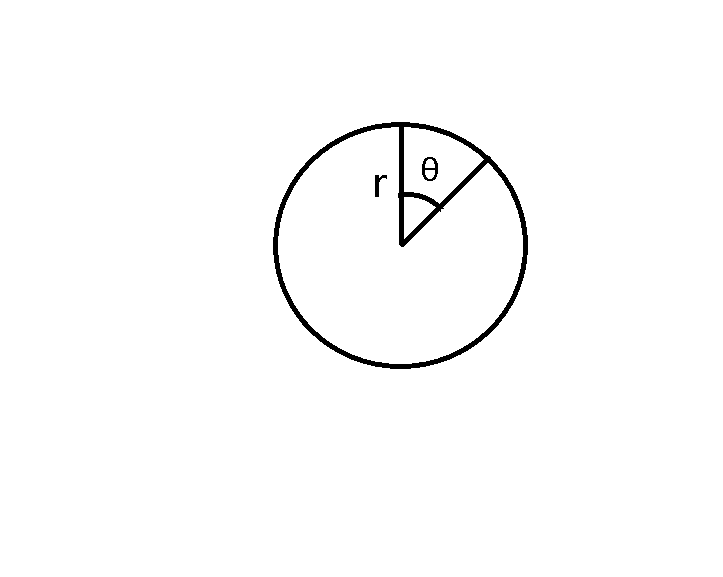# Practice on Toph

Participate in exhilarating programming contests, solve unique algorithm and data structure challenges and be a part of an awesome community.

# Arshiya's Sector

By shajia · Limits 1s, 512 MB

One day Mama came to see Arshiya. Mama knows that Arshiya likes problem solving. So he gave Arshiya an interesting problem. Mama also told Arshiya to think about the problem, and she will find the answer. We all know that how hard-working Arshiya is! She found the answer and now she wants to know that if the problem can be solved by others. So now Mama and Arshiya have arranged a contest and ask you to solve that problem.You are given a circle with a sector. The radius and the angle of that sector of the circle are also given. You have to find the area of that sector.

## Input

The first line of the input will contain an integer $T$ ($0 < T \le 100$), the number of test cases. Then for each test case, there will be two real numbers $R$ ($1 \le R \le 10^2$) and $A$ ($1 \le A \le 360$), the radius of the circle and the angle (in degree) of the sector.

## Output

In the output, print the area of the sector.

The answer is considered correct if the absolute or relative error doesn’t exceed $10^{- 4}$.

## Sample

InputOutput
9
8 56
9 79
5 47
14 102
23 145
10 189
2 210
5 280
4 300
31.276300196
55.841809418
10.253809355
174.463112029
669.377401371
164.933614313
7.330382858
61.086523820
41.887902048

•Geometry

### Statistics

98% Solution Ratio

tutul_hossainEarliest, May '19

tutul_hossainFastest, 0.0s

emrul_muLightest, 0 B

bokaifShortest, 76B

### Submit